# Parse Tree | Derivations | Automata

## Parse Tree-

• The process of deriving a string is called as derivation.
• The geometrical representation of a derivation is called as a parse tree or derivation tree.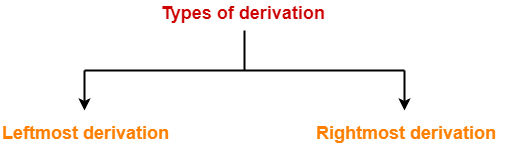## 1. Leftmost Derivation-

• The process of deriving a string by expanding the leftmost non-terminal at each step is called as leftmost derivation.
• The geometrical representation of leftmost derivation is called as a leftmost derivation tree.

### Example-

Consider the following grammar-

S → aB / bA

S → aS / bAA / a

B → bS / aBB / b

(Unambiguous Grammar)

Let us consider a string w = aaabbabbba

Now, let us derive the string w using leftmost derivation.

### Leftmost Derivation-

S → aB

→ aaBB (Using B → aBB)

→ aaaBBB (Using B → aBB)

→ aaabBB (Using B → b)

→ aaabbB (Using B → b)

→ aaabbaBB (Using B → aBB)

→ aaabbabB (Using B → b)

→ aaabbabbS (Using B → bS)

→ aaabbabbbA (Using S → bA)

→ aaabbabbba (Using A → a)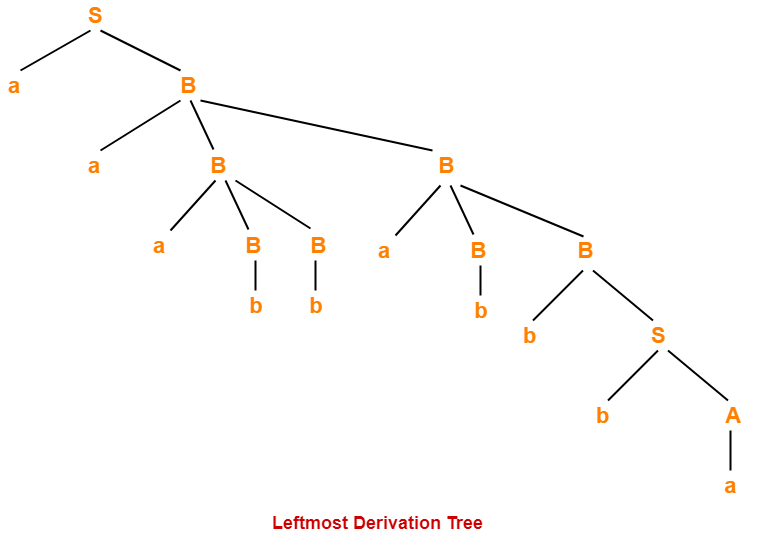## 2. Rightmost Derivation-

• The process of deriving a string by expanding the rightmost non-terminal at each step is called as rightmost derivation.
• The geometrical representation of rightmost derivation is called as a rightmost derivation tree.

### Example-

Consider the following grammar-

S → aB / bA

S → aS / bAA / a

B → bS / aBB / b

(Unambiguous Grammar)

Let us consider a string w = aaabbabbba

Now, let us derive the string w using rightmost derivation.

### Rightmost Derivation-

S → aB

→ aaBB (Using B → aBB)

→ aaBaBB (Using B → aBB)

→ aaBaBbS (Using B → bS)

→ aaBaBbbA (Using S → bA)

→ aaBaBbba (Using A → a)

→ aaBabbba (Using B → b)

→ aaaBBabbba (Using B → aBB)

→ aaaBbabbba (Using B → b)

→ aaabbabbba (Using B → b)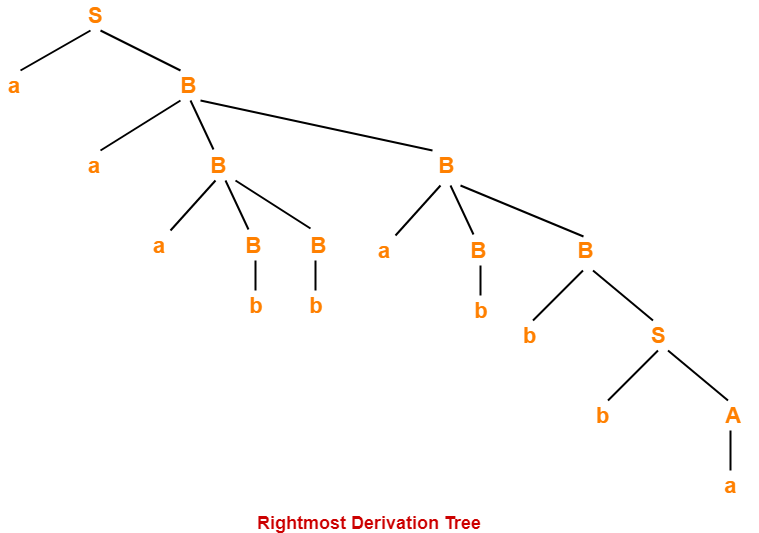### NOTES

• For unambiguous grammars, Leftmost derivation and Rightmost derivation represents the same parse tree.
• For ambiguous grammars, Leftmost derivation and Rightmost derivation represents different parse trees.

Here,

• The given grammar was unambiguous.
• That is why, leftmost derivation and rightmost derivation represents the same parse tree.

 Leftmost Derivation Tree = Rightmost Derivation Tree

## Properties Of Parse Tree-

• Root node of a parse tree is the start symbol of the grammar.
• Each leaf node of a parse tree represents a terminal symbol.
• Each interior node of a parse tree represents a non-terminal symbol.
• Parse tree is independent of the order in which the productions are used during derivations.

## Yield Of Parse Tree-

• Concatenating the leaves of a parse tree from the left produces a string of terminals.
• This string of terminals is called as yield of a parse tree.

## Problem-01:

Consider the grammar-

S → bB / aA

A → b / bS / aAA

B → a / aS / bBB

For the string w = bbaababa, find-

1. Leftmost derivation
2. Rightmost derivation
3. Parse Tree

## Solution-

### 1. Leftmost Derivation-

S → bB

→ bbBB (Using B → bBB)

→ bbaB (Using B → a)

→ bbaaS (Using B → aS)

→ bbaabB (Using S → bB)

→ bbaabaS (Using B → aS)

→ bbaababB (Using S → bB)

→ bbaababa (Using B → a)

### 2. Rightmost Derivation-

S → bB

→ bbBB (Using B → bBB)

→ bbBaS (Using B → aS)

→ bbBabB (Using S → bB)

→ bbBabaS (Using B → aS)

→ bbBababB (Using S → bB)

→ bbBababa (Using B → a)

→ bbaababa (Using B → a)

### 3. Parse Tree-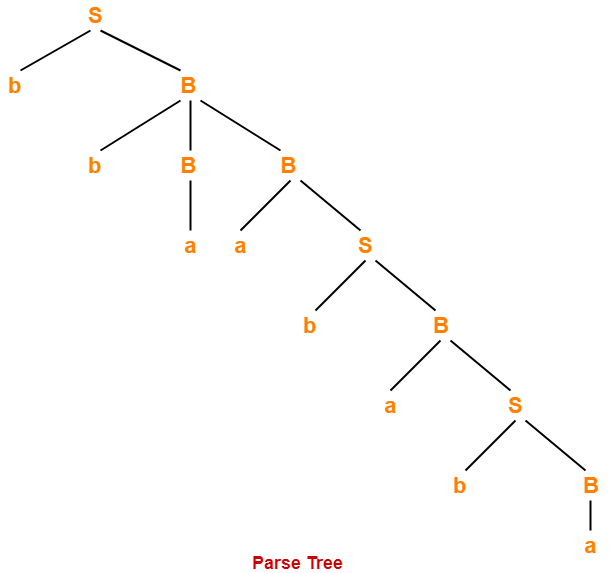• Whether we consider the leftmost derivation or rightmost derivation, we get the above parse tree.
• The reason is given grammar is unambiguous.

## Problem-02:

Consider the grammar-

S → A1B

A → 0A / ∈

B → 0B / 1B / ∈

For the string w = 00101, find-

1. Leftmost derivation
2. Rightmost derivation
3. Parse Tree

## Solution-

### 1. Leftmost Derivation-

S → A1B

→ 0A1B (Using A → 0A)

→ 00A1B (Using A → 0A)

→ 001B (Using A → ∈)

→ 0010B (Using B → 0B)

→ 00101B (Using B → 1B)

→ 00101 (Using B → ∈)

### 2. Rightmost Derivation-

S → A1B

→ A10B (Using B → 0B)

→ A101B (Using B → 1B)

A101 (Using B → ∈)

→ 0A101 (Using A → 0A)

→ 00A101 (Using A → 0A)

→ 00101 (Using A → ∈)

### 3. Parse Tree-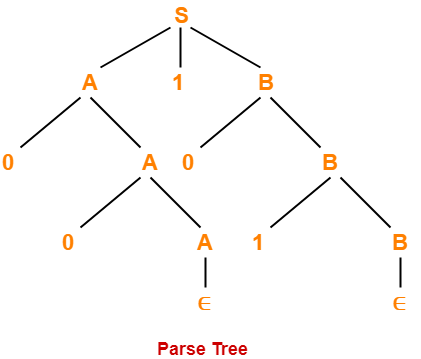• Whether we consider the leftmost derivation or rightmost derivation, we get the above parse tree.
• The reason is given grammar is unambiguous.

To gain better understanding about Derivations and Parse Tree,

Watch this Video Lecture

Next Article- Elimination of Left Recursion

Get more notes and other study material of Theory of Automata and Computation.

Watch video lectures by visiting our YouTube channel LearnVidFun.

SummaryArticle Name
Parse Tree | Derivations | Automata
Description
In automata, derivation is a process of deriving a string. Parse tree or Derivation tree is the geometrical representation of a derivation. Leftmost Derivation and Rightmost Derivation are the two types of derivation.
Author
Publisher Name
Gate Vidyalay
Publisher Logo